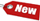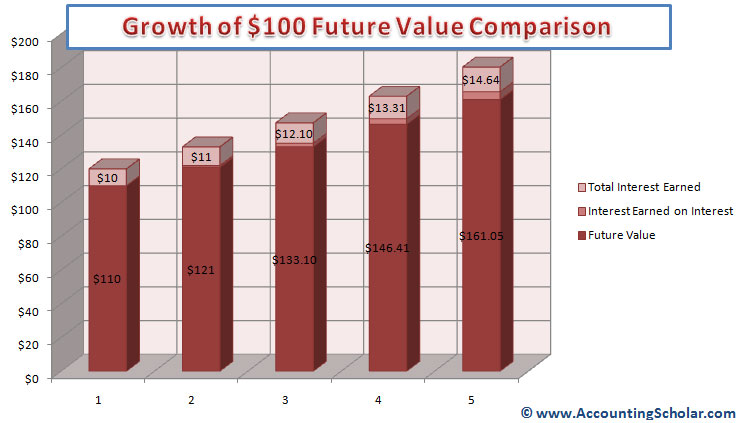Browse Accounting Lessons HereAccounting Terms & DefinitionsAccounting for Merchandising ActivitiesDebits and Credits (Double Entry Accounting)Business Valuation FormulasTime Value of Money & Present/Future ValuesComplex Debt & Equity InstrumentsCommon Stock & Shareholder's EquityAccounting & Finance RatiosValuing Common StockCorporate Income TaxesLower of Cost or Market (LCM) & Inventory ValuationChart of Accounts & BookkeepingBonds Payable & Long Term LiabilitiesCapital AssetsGAAP, Accrual & Cash Accounting, Information Commodity, Internal Controls & MaterialityWhat category of browser are you on this website? Accounting student (homework help) Finance professor (university research) Accounting manager (at work) Other Explore Careers in Accounting and FinanceVisit our section on Careers in Accounting & Finance to explore vast opportunities in this industry.

Chapter 4.2® - Compounding Interest Homework Problem & Time Value of Money Continued - Future Value Formula, Growth of \$100 & Future Value Comparisons

From the introduction to time value of money (part 4.1), we can derive a formula, which will be the future value of \$1 invested for t periods at a rate of r per period. The equation is:

 Future Value = \$1 x (1 + r)t

The expression (1 + r)t is sometimes referred to as the future value interest factor for \$1 invested at r percent for t periods of time.

Let’s use this future value formula to derive the original \$121 at the end of the 2nd year.

 Future Value = \$1 x (1 + r)t Future Value = \$1 x (1+0.1)2 Future Value = \$1 x (1.1)2 Future Value = \$1 x (1.21) Future Value = \$1.21 x \$100 Future Value = \$121 What would be \$100 worth in 5 years, at a 10% interest rate? Future Value = \$1 x (1 + r)t Future Value = \$1 x (1+0.1)5 = 1.15 = 1.61051 Future Value = 1.61051 x \$100 = \$161.05

The table below shows the growth of \$100 each year at 10% interest. Each year, the interest earned is equal to the beginning amount multiplied by the interest rate (10%). How much of this \$161.05 is simple interest, and how much of it is compounding interest? Well \$100 x 0.10 = \$10 per year, so in 5 years, the total simple interest is 5 years x \$10 = \$50. Thus, we can calculate the compounding interest by subtracting \$161.05 - \$150 = \$11.05

 Year (A) Beginning Amount (B) Simple Interest (C) \$100 x 10% Interest Earned on Interest (D) ((B) x 10%) - C Total Interest Earned (E) (C + D) Ending Amount (F) (B + E) 1 \$100 \$10 \$0 \$10 \$110 2 \$110 \$10 \$1 \$11 \$121 3 \$121 \$10 \$2.1 \$12.1 \$133.10 4 \$133.10 \$10 \$3.31 \$13.31 \$146.41 5 \$146.41 \$10 \$4.641 \$14.641 \$161.051The above graph shows the growth of \$100 from inception at year 1 earning simple interest of \$10 (10%) and earning \$11 in the 2nd year, thus making the new total compounded earnings to \$21 at the end of year 2. This compounding effect continues to year 5 when the money has grown from \$100 to \$161.05 in Year 5.

Compound Interest Homework ProblemConsider you found an investment that pays 15% annual interest, which sounds fantastic to you! This makes you invest \$1000 in to it, how much will you have in 3 years? How much will you have in 7 years? And at the end of 7 years, how much interest will you have earned, and how much of it will be compounding interest?
Note: We recommend you try this problem without looking at the solution below first, however if you get stuck, feel free to take a peak!

 a) Future Value = \$1 x (1 + r)t = =\$1 x (1 + 0.15)3 = \$1.153 = 1.520875 x \$1000 = \$1,520.875 b) Future Value = \$1 x (1 + r)t = =\$1 x (1 + 0.15)7 = \$1.157 = 2.66002 x \$1000 = \$2,660.02

You invested \$1000 x 15% = \$150 simple interest each year, thus total simple interest in 7 years = \$150 x 7 = \$1,050

 Compound Interest = Total Interest – Simple Interest Compound Interest = \$1,660.02 -\$1050 Compound Interest = \$610.02